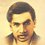# $e-2=1$

We know that:

$\frac 1{2!}+\frac 1{3!}+\frac 1{4!}+\frac 1{5!}+\frac 1{6!}+\cdots =e-2\approx0.71828$

But I am getting the above sum as 1 , as shown below:

\begin{aligned}S & = \frac 1{2!}+\frac 1{3!}+\frac 1{4!}+\frac 1{5!}+\frac 1{6!}+\cdots \\ & = \frac 1{2!} + \frac {3-2}{3!} +\frac {4\times2-7}{4!}+\frac {5\times7-34}{5!}+\frac {6\times34-203}{6!}+\cdots \\ & = \frac 1{2!}+\frac 1{2!}-\frac 2{3!}+\frac 2{3!}-\frac 7{4!}+\frac 7{4!}-\frac {34}{5!}+\frac {34}{5!}-\frac {203}{6!}+\cdots \\ & = 1 \end{aligned}Note by Mrigank Shekhar Pathak
3 years, 7 months ago

This discussion board is a place to discuss our Daily Challenges and the math and science related to those challenges. Explanations are more than just a solution — they should explain the steps and thinking strategies that you used to obtain the solution. Comments should further the discussion of math and science.

When posting on Brilliant:

• Use the emojis to react to an explanation, whether you're congratulating a job well done , or just really confused .
• Ask specific questions about the challenge or the steps in somebody's explanation. Well-posed questions can add a lot to the discussion, but posting "I don't understand!" doesn't help anyone.
• Try to contribute something new to the discussion, whether it is an extension, generalization or other idea related to the challenge.

MarkdownAppears as
*italics* or _italics_ italics
**bold** or __bold__ bold
- bulleted- list
• bulleted
• list
1. numbered2. list
1. numbered
2. list
Note: you must add a full line of space before and after lists for them to show up correctly
paragraph 1paragraph 2

paragraph 1

paragraph 2

[example link](https://brilliant.org)example link
> This is a quote
This is a quote
    # I indented these lines
# 4 spaces, and now they show
# up as a code block.

print "hello world"
# I indented these lines
# 4 spaces, and now they show
# up as a code block.

print "hello world"
MathAppears as
Remember to wrap math in $$ ... $$ or $ ... $ to ensure proper formatting.
2 \times 3 $2 \times 3$
2^{34} $2^{34}$
a_{i-1} $a_{i-1}$
\frac{2}{3} $\frac{2}{3}$
\sqrt{2} $\sqrt{2}$
\sum_{i=1}^3 $\sum_{i=1}^3$
\sin \theta $\sin \theta$
\boxed{123} $\boxed{123}$

Sort by:

The absolute value of each term of the alternating series approaches $3-e$ rather than zero. So, strangely enough, the new series does not converge. In particular, in any finite version, you are left with $(\sum_{k=4}^n \frac{1}{k!})-\frac{2}{3!}$ as a final term that does not cancel with anything, which approaches $e-3$.

- 3 years, 7 months ago

I used the recurrence relation $a_{n+1}=na_n-1$, $a_3=2$ to describe the numerators of the fractions. And then used the method from this wikipedia page (https://en.wikipedia.org/wiki/Recurrencerelation#Solvingfirst-ordernon-homogeneousrecurrencerelationswithvariablecoefficients) to get the formula. The limit of the absolute value of the terms ultimately simplifies to $3-e$.

- 3 years, 7 months ago

Although you would have to show $e\neq 3$.

- 3 years, 7 months ago

But I think the flaw should be clear...

- 3 years, 7 months ago

\begin{aligned}S &= \frac 1{2!}+\frac 1{3!}+\frac 1{4!}+\frac 1{5!}+\frac 1{6!}+\cdots \\ &= \frac 1{2!} + \frac {3-2}{3!} +\frac {4\times2-7}{4!}+\frac {5\times7-34}{5!}+\frac {6\times34-203}{6!}+\cdots \\ & = \frac 1{2!}+\frac 1{2!}-\frac 2{3!}+\frac 2{3!}-\frac 7{4!}+\frac 7{4!}-\frac {34}{5!}+\frac {34}{5!}-\frac {203}{6!}+\cdots \\ & = 1-\lim_{n\rightarrow \large \infty} \dfrac{a_{n}}{n!}\\\\ \text{Where } &a_{n} \text{ is given by the reccurance,}\\ a_{n}&=na_{n-1}-1\\\\ \text{I was } &\text{able to simplify } a_{n} \text{ as,}\\ a_n&=3(n!)-\sum_{m=0}^n \dfrac{n!}{(n-m)!}\\\\ \implies S&=1-\lim_{n\rightarrow \large \infty} \dfrac{3(n!)-\sum_{m=0}^n \dfrac{n!}{(n-m)!}}{n!}\\\\ &=1-3+\lim_{n\rightarrow \large \infty} \dfrac{\sum_{m=0}^n \dfrac{n!}{(n-m)!}}{n!}\\\\ &=-2+\lim_{n\rightarrow \large\infty}\sum_{m=0}^n \dfrac{1}{(n-m)!}\\ &\text{setting } (n-m) =t\\ &=-2+\lim_{n\rightarrow \large\infty}\sum_{t=0}^n \dfrac{1}{(t)!}\hspace{5mm} \color{#3D99F6}\text{t varies from } 0 \text{ to } n\\ &=\color{#EC7300}\boxed{\color{#333333}e-2}\end{aligned}

- 3 years, 7 months ago

What you have done is excellent , but it would be better if you show that $\displaystyle 2<\lim_{n\to \infty}\sum_{t=0}^{n}\dfrac 1{t!}<3$ thus, $S=e-2<1$

- 3 years, 7 months ago

Let the general term be $\dfrac {nl_{n-1}-l_n}{n!}$. You are assuming that $\dfrac {l_n}{n!}$ converges as $n \to \infty$. In your example you start splitting with the $3!$ term, you will get another value if you start with $4!$ term. It cannot be the answer.

- 3 years, 7 months ago

please explain in detail through some example, I can't understand why it would be wrong. Suppose I leave the $3!$ term and split the remaining terms in the same way then I am getting the same answer (i.e., 1)

- 3 years, 7 months ago

You are assuming your procedure converges. It does not converge so you don't get the answer. I thought if you start splitting at $4!$ term you will get $\dfrac 1{2!} + \dfrac 1{3!} + \dfrac 1{3!} = \dfrac 56$.

- 3 years, 7 months ago

could you please show with some test that the procedure does not converge

- 3 years, 7 months ago

No. It is tough.

- 3 years, 7 months ago

You may have a look at Mathematics Stack Exchange it has got a good discussion on it.

- 3 years, 7 months ago